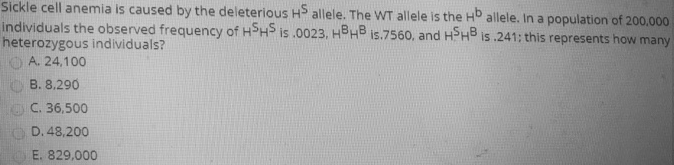# Problem: Sickle cell anemia is caused by the deleterious HS allele. The WT allele is the HB allele. In a population of 200,000 individuals, the observed frequency of HSHS is 0.0023, HBHB is 0.7560, and HSHB is 0.241. How many are the heterozygous individuals?A. 24,100B. 8,290C. 36,500D. 48,200E. 829,200

###### FREE Expert Solution

The given problem shows that the different genotypic frequencies for the trait: 0.0023 for HSHS, 0.7560 for HBHB, and 0.241 for HSHB. In a Hardy-Weinberg equilibrium, the frequency of the dominant allele can be represented by p, and the frequency of the recessive allele can be represented by q. For a trait that only has two alleles, p + q = 1. Furthermore, these values can be used to compute for the genotypic frequencies: p2 is for the homozygous dominant, 2pq is for the heterozygotes and q2 for the homozygous recessive.###### Problem Details

Sickle cell anemia is caused by the deleterious HS allele. The WT allele is the HB allele. In a population of 200,000 individuals, the observed frequency of HSHS is 0.0023, HBHB is 0.7560, and HSHB is 0.241. How many are the heterozygous individuals?

A. 24,100

B. 8,290

C. 36,500

D. 48,200

E. 829,200